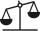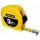We need two tenths kg of a carrot, one tenth of peas and three tenths of of tomatoes to make salad. Express the fraction of the weight of the vegetables to be salad. Convert the result to grams.

Result

f =  0.6 kg
g =  600 g

Solution:Leave us a comment of this math problem and its solution (i.e. if it is still somewhat unclear...):Be the first to comment!To solve this verbal math problem are needed these knowledge from mathematics:

Need help calculate sum, simplify or multiply fractions? Try our fraction calculator. Do you want to convert mass units?

Next similar math problems:

1. RecipeA recipe requires 2 pounds of flour. If a chef wants to triple the recipe, how many ounces of flour will be needed?
2. Fraction and a decimalWrite as a fraction and a decimal. One and two plus three and five hundredths
3. Pound2kilosHow many pounds make 1 kilograms?
4. In fractionsAn ant climbs 2/5 of the pole on the first hour and climbs 1/4 of the pole on the next hour. What part of the pole does the ant climb in two hours?
5. Mixed2improperWrite the mixed number as an improper fraction. 166 2/3
6. Fraction to decimalWrite the fraction 3/22 as a decimal.
7. Lengths of the poolMiguel swam 6 lengths of the pool. Mat swam 3 times as far as Miguel. Lionel swam 1/3 as far as Miguel. How many lengths did mat swim?
8. ZdeněkZdeněk picked up 15 l of water from a 100-liter full-water barrel. Write a fraction of what part of Zdeněk's water he picked.
9. NotebooksLiza a store owner buys 560 notebooks. He sold 3/8 of the notebook then she adds the stocks of notebook of 1/4 of the number of notebooks she bought. What is the total number of notebook she bought?
10. Sum of fractionsWhat is the sum of 2/3+3/5?
11. Adding mixed numerals3 3/4 + 2 3/5 + 5 1/2 Show your solution.
12. 3 children3 children had to divide 4 pounds is candy. How much candy did each child get?
13. BrickIsosceles scale has on one side all brick and second weight 1 kg and 1/4 of brick. The balance is in equilibrium. What is the weight of a brick?
14. Adding mixed numbersAdd this two mixed numbers: 1 5/6 + 2 2/11=
15. Conversion of unitsComplete the following length data
16. Roses and tulipsAt the florist are 50 tulips and 5 times less roses. How many flowers are in flower shop?
17. Addition of mixed numeralsAdd two mixed fractions: 2 4/6 + 1 3/6